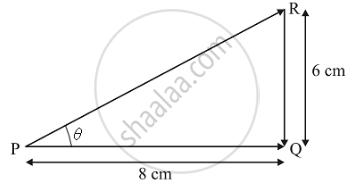Share

# An Ant Travels a Distance of 8 Cm from P to Q and Then Moves a Distance of 6 Cm at Right Angles to Pq. Find Its Resultant Displacement. - CBSE Class 9 - Science

ConceptDistance and Displacement

#### Question

An ant travels a distance of 8 cm from P to Q and then moves a distance of 6 cm at right angles to PQ. Find its resultant displacement.

#### Solution

We have to find the resultant displacement from the given diagram :We have:
PQ = 8 cm and QR = 6 cm
Resultant displacement :
PR = sqrt(PQ^2 + QR^2)
= sqrt(64+36)
= sqrt(100)
= 10 cm
The direction of this displacement is from P to R. If θ is the angle made by PR with PQ then,'
tan  θ = "RQ"/"PQ"
⇒ tan θ = 36/64
⇒ θ = tan^-1 0.5625
⇒ θ = 29.36^circ
This is the angle made by the resultant with PQ .

Is there an error in this question or solution?

#### Video TutorialsVIEW ALL 

Solution An Ant Travels a Distance of 8 Cm from P to Q and Then Moves a Distance of 6 Cm at Right Angles to Pq. Find Its Resultant Displacement. Concept: Distance and Displacement.
S